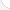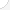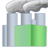Computer control programmers and operatorsDescription: These workers use special computer-controlled machines to cut and shape products such as car parts, machine parts, and compressors. They use lathes, spindles, and milling machines following blueprints from engineers. While they work they must constantly monitor readouts from the computer to make sure parts are being made properly. Because of the machinery they use, this can be a dangerous job.Source: Bureau of Labor Statistics, U.S. Department of Labor, Occupational Outlook Handbook, 2008-09 Edition at http://www.bls.gov/OCO/Complete Job Profile: http://www.bls.gov/oco/ocos286.htmSalary: \$28,001 to \$50,000 per yearComments:

There are 41 math topics Computer control programmers and operators need to know.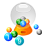Basic Math / Algebra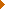FractionsDecimalsRatio and ProportionPercentCustomary MeasurementMetric MeasurementMeasurement ConversionBasic ProbabilityBasic StatisticsStatistical GraphingNegative NumbersBasic Problem Solving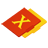First-Year AlgebraUsing FormulasLinear EquationsLinear InequalitiesOperations with PolynomialsFactoring PolynomialsRational ExpressionsCoordinate Graphing 2DLinear SystemsRadicalsQuadratic EquationsAlgebraic Representation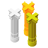Second-Year Algebra / TrigonometryFunctionsVariationSequences and SeriesMatricesCoordinate Graphing 3DAdvanced StatisticsPolar Coordinates/GraphsVectors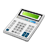Other TopicsCalculus and Higher MathBasic Calculator UseScientific Calculator UseComputer UseComputer ProgrammingGroup Problem SolvingMental MathInductive/Deductive ReasoningMath CommunicationsMathematical Modeling